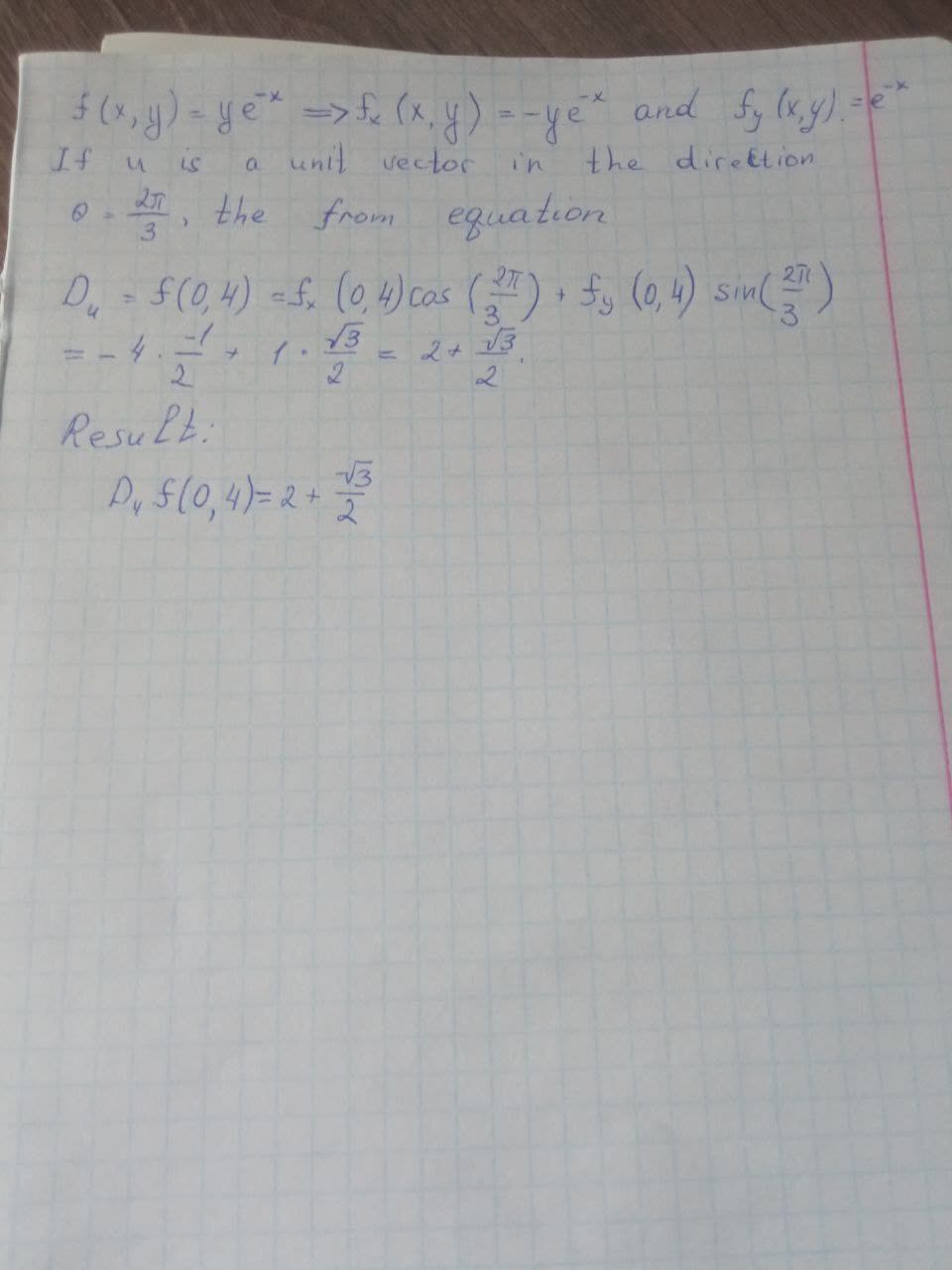# Find the directional derivative of f at the given point in the direction indicated by the angle theta. f(x,y)=ye^{-x}, (0,4), \theta=\frac{2\pi}{3}ossidianaZ 2021-09-18 Answered
Find the directional derivative of f at the given point in the direction indicated by the angle theta. $f\left(x,y\right)=y{e}^{-x},\left(0,4\right),\theta =\frac{2\pi }{3}$
You can still ask an expert for help

• Questions are typically answered in as fast as 30 minutes

Solve your problem for the price of one coffee

• Math expert for every subject
• Pay only if we can solve itrogreenhoxa8
Now we have: